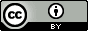## Wednesday, January 15, 2014

### EFMO-PCM: A roadmap. Version 2

Having read the EFP-PCM interface more carefully again I need to make a major update of a previous post.  Since Blogger doesn't have version numbers, here comes a separete post.

One of the things still missing in the EFMO method is an interface to PCM.  Here I attempt to sketch the method, based on the FMO-PCM and EFP-PCM interfaces.

The EFMO-PCM electrostatic interaction free energy between solute and solvent plus the polarization energy is

$G_{pol+sol}=-\frac{1}{2}\mathbf{F}^{mul} \boldsymbol{\mu}^T+\frac{1}{2}\mathbf{V}^{mul} \mathbf{q}^T$

As Hui and Mark wrote:

"The interaction between the induced dipoles and charges is implicitly described with the matrices $\mathbf{R}$ and $\mathbf{R}^T$, just as the interaction between the EFP induced dipoles is implicitly described with the off-diagonal elements of matrix $\mathbf{D}$, and the interaction between the PCM induced charges is implicitly described with the off-diagonal elements of matrix $\mathbf{C}$"

$\mathbf{q}$ are the apparent surface charges (ASCs), which for large systems are obtained by solving this equation iteratively

$\mathbf{Cq}=-\mathbf{V}$

$\mathbf{V}$ is the electrostatic potential from the static multipoles and induced dipoles, respectively:

$\mathbf{V=V^{\text{mul}}+V^{\mu}}$

The simplest approximation to $\mathbf{V}$ is
$$\mathbf{V} =\sum_I^N \mathbf{V}_{I} = \sum_I^N (\mathbf{V}^{\text{mul}}_{I} +\mathbf{V}^{\mu}_{I})$$
The potential at tesserae $j$ due to induced dipoles on fragment $I$ is given by:
$$\mathbf{V}_I^\mu (j)=\sum_{i \in I} (\mathbf{R}^T)_{ji}\boldsymbol{\mu_{i}}$$
The induced dipoles are obtained iteratively:
$$\boldsymbol{\mu}_i=\boldsymbol{\alpha}_i\left(\mathbf{F}^{\text{mul}}_i+\mathbf{F}^q_i-\sum_{i\neq j}\mathbf{D}_{ij}\boldsymbol{\mu}_j\right)$$
where $\boldsymbol{\alpha}_i$ is the dipole polarizability tensor at site $i$ and $\mathbf{F}^{\text{mul}}_i$ and $\mathbf{F}^q_i$ are the electrostatic fields due to all static multipoles and ASCs felt at point $i$.

Procedure:

1. Compute EFMO gas phase energy

2. Use gas phase static multipoles and $\boldsymbol{\mu}$ to construct $\mathbf{V}$

3. Solve $\mathbf{Cq=-V}$

4. Use $\mathbf{q}$ and $\boldsymbol{\mu}_i=\boldsymbol{\alpha}_i\left(\mathbf{F}^{\text{mul}}_i+\mathbf{F}^q_i-\sum_{i\neq j}\mathbf{D}_{ij}\boldsymbol{\mu}_j\right)$ to find new $\boldsymbol{\mu}$

5. Repeat steps 2-4 until self consistency

6. Compute $G_{pol+solv}$

Gradient

$$G_{pol+sol}^x=G_{sol}^x+G_{pol}^x$$
$$G_{sol}^x=\mathbf{V}^x \mathbf{q}^T+\frac{1}{2}\mathbf{q}^T\mathbf{C}^x\mathbf{q}$$
$$G_{pol}^x=-\mathbf{F}^{mul,x} \boldsymbol{\mu}^T+\boldsymbol{\mu}^T\mathbf{D}^x\boldsymbol{\mu}$$

The most tricky part is $\mathbf{V}^{\mu,x} \mathbf{q}^T$:

$$\sum_n^N V_n^{\mu,x} q_n = \sum_i^I V_i^{\mu,B,x_i} q_i+ \sum_j^J V_j^{\mu,A,x_A} q_j$$
$$V_i^{\mu ,B,{x_i}} = \sum\limits_{m \ne A}^{} {{{\left( {\frac{{ - ({{\bf{r}}_i} - {{\bf{r}}_m})}}{{{{\left| {{{\bf{r}}_i} - {{\bf{r}}_m}} \right|}^3}}}} \right)}^{{x_i}}}} \boldsymbol{\mu}_m^B$$

$$V_j^{\mu,A,{x_A}} = {\left( {\frac{{ - ({{\bf{r}}_j} - {{\bf{r}}_A})}}{{{{\left| {{{\bf{r}}_j} - {{\bf{r}}_A}} \right|}^3}}}} \right)^{{x_A}}}\boldsymbol{\mu}^A$$

Here $n$ sum over the all the tesserae ($N$).  The tesserae set $I$ belong to the sphere centered on atom $A$ - the atoms whose coordinate ($x_A = x$) we are taking the derivative wrt. $x_i$ refers to the coordinate of a ASC associated with a $I$ tessera.  $J$ are all other tessera belonging to atoms collectively referred to as $B$.  Notice that the gradient involving tessera and induced dipoles belonging to the same atom is zero, $V_i^{\mu,A,x_A}=0$

The tricky thing in general with the EFMO gradient involving induced dipoles is that they are not centered on atoms. In text S1 of this paper we describe how we deal with this for bond-dipoles, $$V_j^{\mu,A,{x_A}}=f(R)V_j^{\mu,A,{x_{LMO-A}}}$$but we don't talk about how to do it for lone pairs.  Perhaps we simply set $x_A = x_{LMO-A}$?  Anyway, it should only be an issue for $V_j^{\mu,A,{x_A}}$.

Miscellaneous
For more accurate results one can approximate $\mathbf{V}$ as
$$\mathbf{V}=\sum_I^N \mathbf{V}_{I}+\sum^N_{I}\sum^N_{J<I} (\mathbf{V}_{IJ}-\mathbf{V}_{I}-\mathbf{V}_{J})$$
This essentially means that the gas phase static multipoles and $\alpha$'s are corrected in step 2. E.g. for static monopoles ($q$)'s:
$$V_I^q(i)=\frac{q_i^{I}}{|r-r_i|}$$
and
\begin{align*} V(i)&=V_I^q(i)+\sum^N_{J<I} (V_{IJ}^q(i)-V_I^q(i))\\ & = [q^{I}_i+\sum^N_{J<I}(q^{IJ}_i-q^{I}_i)]\frac{1}{|r-r_i|} \\ \end{align*}
All other steps are the same.

A tip on dealing with complex papers
I found the EFP-PCM paper a big mouthful and had to revert to an old trick that I'll share with you here.  Mainly what makes the paper complex is its length so that definitions and equations are pages apart.  For such papers I usually fire up PowerPoint and Snapndrag (for screenshots) and grab what I think are the essential pieces, rearrange some, and add "missing equations" when needed.  You can see the final result here (the first two pages are the main results and the rest are "derivations").

I highly recommend this approach for complex papers or making sense of a collection of papers on a specific topic.This work is licensed under a Creative Commons Attribution 4.0